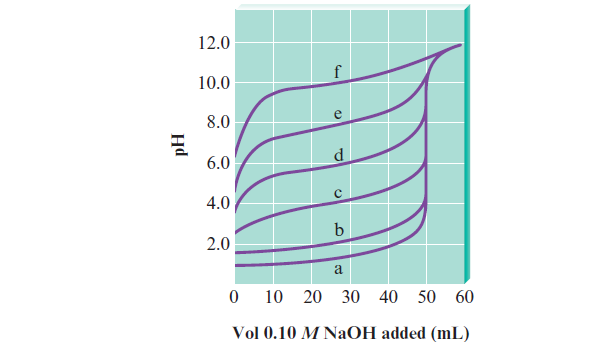# Problem: The following plot shows the pH curves for the titrations of various acids by 0.10 M NaOH (all of the acids were 50.0-mL samples of 0.10 M concentration).b. Which pH curve corresponds to the strongest acid? Which point on the pH curve would you examine to see if this acid is a strong acid or a weak acid (assuming you did not know the initial concentration of the acid)?

###### FREE Expert Solution
96% (416 ratings)
###### Problem Details

The following plot shows the pH curves for the titrations of various acids by 0.10 M NaOH (all of the acids were 50.0-mL samples of 0.10 M concentration).b. Which pH curve corresponds to the strongest acid? Which point on the pH curve would you examine to see if this acid is a strong acid or a weak acid (assuming you did not know the initial concentration of the acid)?